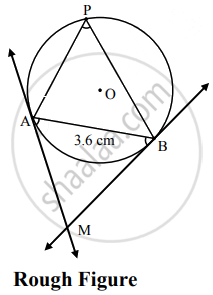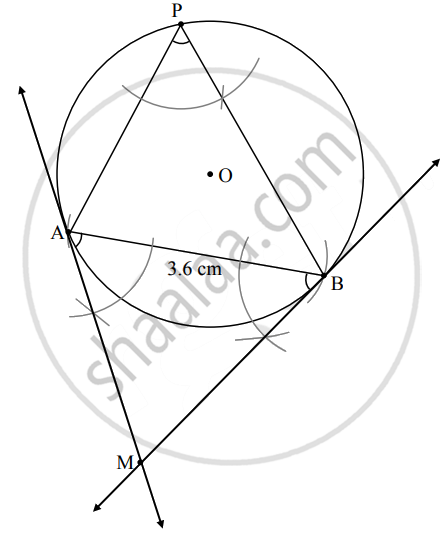# Draw any circle with radius greater than 1.8 cm and less than 3 cm. Draw a chord AB 3.6 cm long in this circle. Tangent to the circle passing through A and B without using the center of the circle - Geometry

Diagram

Draw any circle with radius greater than 1.8 cm and less than 3 cm. Draw a chord AB 3.6 cm long in this circle. Tangent to the circle passing through A and B without using the center of the circle

#### SolutionSteps of construction:

1. Draw a circle of radius 2.5 cm.
2. Draw a chord AB of length 3.6 cm.
3. Take a point P on the major arc, other than A and B.
4. Join AP and BP.
5. Using A and B as vertices and chord AB as one side, draw ∠MAB and ∠MBA equal to ∠APB.
6. Lines containing the rays AM and BM are the tangents to the circle at A and B respectively.
Concept: Construction of a Tangent to the Circle at a Point on the Circle
Is there an error in this question or solution?

Share